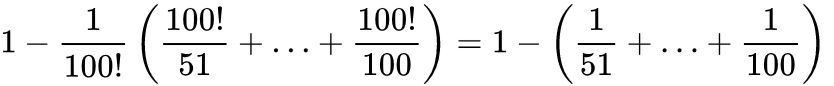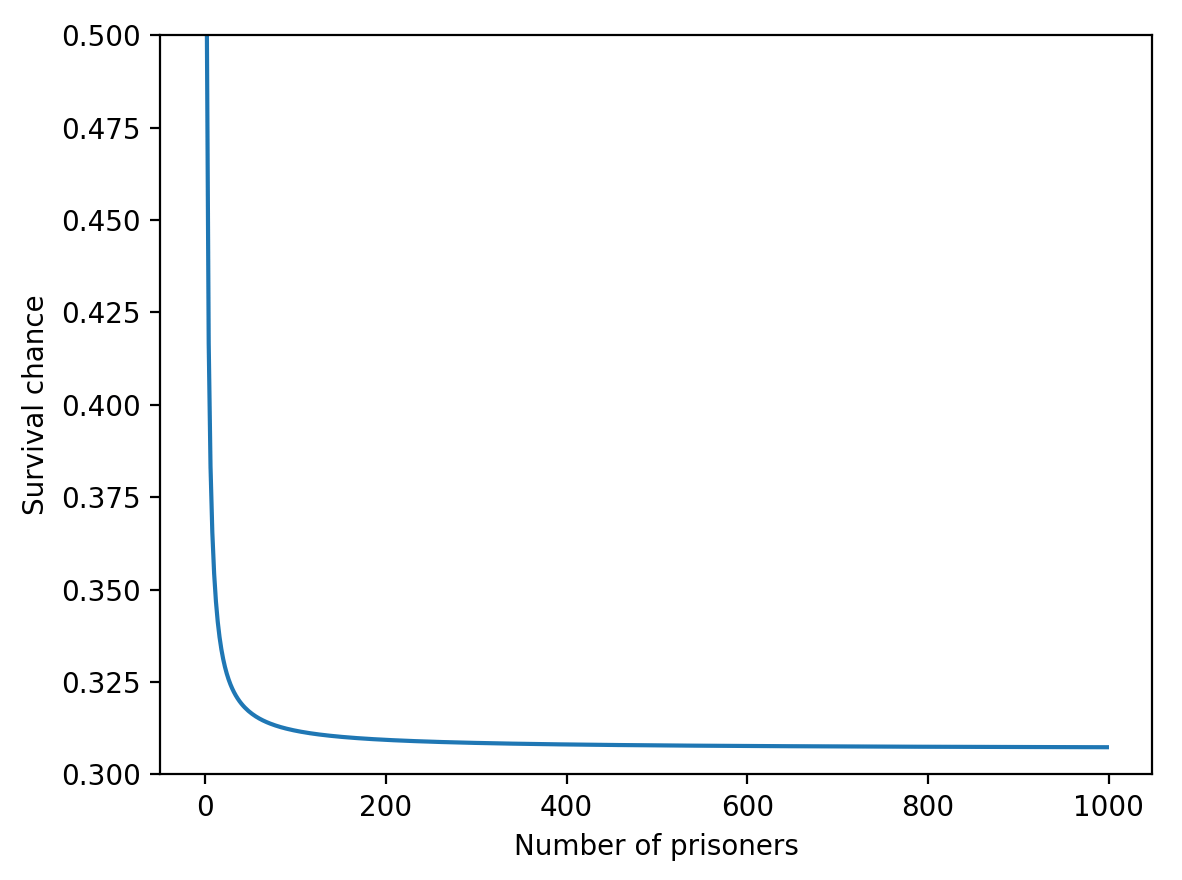# 100 prisoners problem

I’m in the mood for puzzles, so here’s another one. This one is so good that it deserves its own post.

The setup (from wiki):

The director of a prison offers 100 death row prisoners, who are numbered from 1 to 100, a last chance. A room contains a cupboard with 100 drawers. The director randomly puts one prisoner’s number in each closed drawer. The prisoners enter the room, one after another. Each prisoner may open and look into 50 drawers in any order. The drawers are closed again afterwards.

If, during this search, every prisoner finds his number in one of the drawers, all prisoners are pardoned. If just one prisoner does not find his number, all prisoners die. Before the first prisoner enters the room, the prisoners may discuss strategy—but may not communicate once the first prisoner enters to look in the drawers. What is the prisoners’ best strategy?

Suppose that each prisoner selects 50 at random, and don’t coordinate with one another. Then the chance that any particular prisoner gets their own number is 50%. This means that the chance that all 100 get their own number is 1/2¹⁰⁰.

Let me emphasize how crazily small this is. 1/2¹⁰⁰ is 1/1,267,650,600,228,229,401,496,703,205,376; less than one in a decillion. If there were 100 prisoners trying exactly this setup every millisecond, it would take them 40 billion billion years to get out alive once. This is 3 billion times longer than the age of the universe.

Okay, so that’s a bad strategy. Can we do better?

It’s hard to imagine how… While the prisoners can coordinate beforehand, they cannot share any information. So every time a prisoner comes in for their turn at the drawers, they are in exactly the same state of knowledge as if they hadn’t coordinated with the others.

Given this, how could we possibly increase the survival chance beyond 1/2¹⁰⁰?

(…)

(Try to answer for yourself before continuing)

(…)

Let’s consider a much simpler case. Imagine we have just two prisoners, two drawers, and each one can only open one of them. Now if both prisoners choose randomly, there’s only a 1 in 4 chance that they both survive.

What if they agree to open the same drawer? Then they have reduced their survival chance from 25% to 0%! Why? Because by choosing the same drawer, they either both get the number 1, or they both get the number 2. In either case, they are guaranteed that only one of them gets their own number.

So clearly the prisoners can decrease the survival probability by coordinating beforehand. Can they increase it?

Yes! Suppose that they agree to open different drawers. Then this doubles their survival chance from 25% to 50%. Either they both get their own number, or they both get the wrong number.

The key here is to minimize the overlap between the choices of the prisoners. Unfortunately, this sort of strategy doesn’t scale well. If we have four prisoners, each allowed to open two drawers, then random drawing gives a 1/16 survival chance.

Let’s say they open according to the following scheme: 12, 34, 13, 24 (first prisoner opens drawers 1 and 2, second opens 3 and 4, and so on). Then out of the 24 possible drawer layouts, the only layouts that work are 1432 and 3124:

1234 1243 1324 1342 1423 1432
2134 2143 2314 2341 2413 2431
3124 3142 3214 3241 3412 3421
4123 4132 4213 4231 4312 4321

This gives a 1/12 chance of survival, which is better but not by much.

What if instead they open according to the following scheme: (12, 23, 34, 14)?

1234 1243 1324 1342 1423 1432
2134 2143 2314 2341 2413 2431
3124 3142 3214 3241 3412 3421
4123 4132 4213 4231 4312 4321

Same thing: a 1/12 chance of survival.

Scaling this up to 100 prisoners, the odds of survival look pretty measly. Can they do better than this?

(…)

(Try to answer for yourself before continuing)

(…)

It turns out that yes, there is a strategy that does better at ensuring survival. In fact, it does so much better that the survival chance is over 30 percent!

Take a moment to boggle at this. Somehow we can leverage the dependency induced by the prisoners’ coordination to increase the chance of survival by a factor of one decillion, even though none of their states of knowledge are any different. It’s pretty shocking to me that this is possible.

Here’s the strategy: Each time a prisoner opens a drawer, they consult the number in that drawer to determine which drawer they will open next. Thus each prisoner only has to decide on the first drawer to open, and all the rest of the drawers follow from this. Importantly, the prisoner only knows the first drawer they’ll pick; the other 49 are determined by the distribution of numbers in the drawers.

We can think about each drawer as starting a chain through the other drawers. These chains always cycle back into the starting number, the longest possible cycle being 100 numbers and the shortest being 1. Now, each prisoner can guarantee that they are in a cycle that contains their own number by choosing the drawer corresponding to their own number!

So, the strategy is that Prisoner N starts by choosing Drawer N, looking at the number within, then choosing the drawer labeled with that number. Repeat 50 times per each prisoner.

The wiki page has a good description of how to calculate the survival probability with this strategy:

The prison director’s assignment of prisoner numbers to drawers can mathematically be described as a permutation of the numbers 1 to 100. Such a permutation is a one-to-one mapping of the set of natural numbers from 1 to 100 to itself. A sequence of numbers which after repeated application of the permutation returns to the first number is called a cycle of the permutation. Every permutation can be decomposed into disjoint cycles, that is, cycles which have no common elements.

In the initial problem, the 100 prisoners are successful if the longest cycle of the permutation has a length of at most 50. Their survival probability is therefore equal to the probability that a random permutation of the numbers 1 to 100 contains no cycle of length greater than 50. This probability is determined in the following.

A permutation of the numbers 1 to 100 can contain at most one cycle of length. There are exactlyways to select the numbers of such a cycle. Within this cycle, these numbers can be arranged inways since there arepermutations to represent distinct cycles of lengthbecause of cyclic symmetry. The remaining numbers can be arranged inways. Therefore, the number of permutations of the numbers 1 to 100 with a cycle of lengthis equal toThe probability, that a (uniformly distributed) random permutation contains no cycle of length greater than 50 is calculated with the formula for single events and the formula for complementary events thus given by